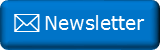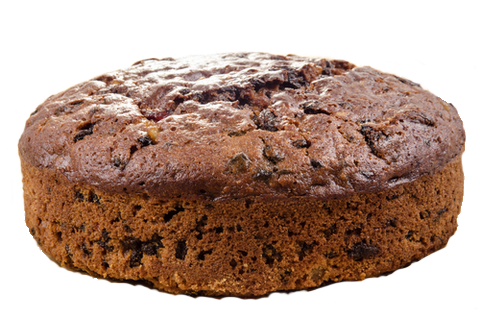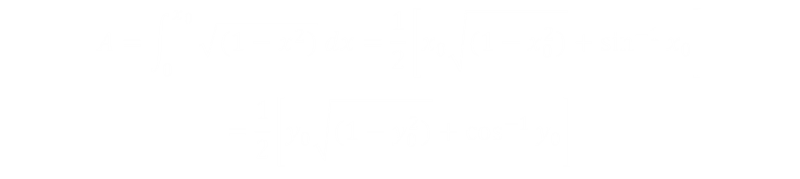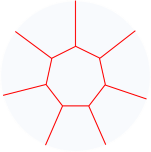﻿ Cake cutting part 2Newsletter: Click here to join an email list and be contacted when a new article is published.      Advertisement

# Cake cutting revisited

Sometimes you rush to get an article out, and in haste make some assumptions. Last week was one of those weeks for me!I published an article last week about the optimal way to slice a cake into eight equal sized pieces using just four straight cuts. A few of my readers pointed out that, technically, yes, if you consider a cut needing to be from edge to edge, the solution I proposed (shown below right) is correct and the optimal solution. However, if you remove the restriction that each cut needs to go edge to edge, and thus allow for more than four cuts, there are ways to do better.If you remove the restriction that each cut needs to go from edge to edge you can do better.

## Better Quarter Cuts

We can do better at the quarter cut stage.

When talking about bisecting the quarter cake I made the (incorrect) assumption that the vertical cut would be the shortest. It’s not. (This does not affect the ultimate solution as, due to symmetry, two quarter bisected pieces can be aligned so that one cut goes through both quarters).

Phil Jackson educated me about my error, and brought to my attention a similar article on math.stackexchange about how to divide a Quarter Circle Pasture into two equal pieces using the shortest fence. It's possible to angle the cut so that it hits the circle a little further down, and is made up by sliding the intersection in the opposite way.

There is an excellent response on that page from a prolific poster with the handle joriki. Here’s a summary of his response:Above left is the cut. To work out the area, we can first work out the area of the vertical part the coordinate (x0, y0), which is the integral of the area under the curve (middle image) as we did in the last article for the vertical cut.Next we need to subtract away the area of the triangle (right image). If we define the slope of the cut to be 1/a, then the formula for the shape is given below. To bisect the quarter we need the resultant area to be an eighth of a circle. For a normalized circle this is π/8.Joriki crunched this formula with Wolfram Alpha, and determined that minimum length of the cut was ≈ 0.887, which is indeed a little shorter than the vertical ≈ 0.915 (about a 3% reduction). This occurs at x0 ≈ 0.519, and y0 ≈ 0.855

Here it is plotted in WolframAlpha.

Because this cut is at an angle, we can't run across two corner pieces with the same straight cut, but it is possible to use the cut, and a mirror of it, to carve the cake into eight pieces. This requires two extra cuts, but reduces the overall cut length to ≈ 7.55 units, a reduction of 1.4% from the four straight cut solution.The optimal solution using this new strategy is plotted above left.

## Better StillIf we are allowed to make as many straight line cuts as we want, we can do better still. First we can make a center piece of a regular heptagon. The area of this shape should be one eighth of the area of the circle. Next, we'll make cuts from each of the vertices to the edge of the cake. With symmetery, these edge pieces will share the remaining 7/8th of the cake to make each piece 1/8th. Thanks for encouraging me to write this up Corey Plover.

The formula for the area of regular polygon is given below (where n is the number of sides). We can invert this to determine the value for the radius of the heptagon (the distance from the centroid to the vertex) based on a normalized circle.The length of each of the spurs cuts from a vertex is 1-r ≈ 0.621, and there are seven in total.

Finally we need to find length of the side of the heptagon. The formula for this is below. Again, there are seven of these cuts.Adding up both sets of seven cuts results in a total length of ≈ 6.65 units.This is a reduction of approximately 16.9% from the sector cut, and just over one unit less total cut length than the four cut solution I described last week. If you look closely you will see this shape is very similar the shape of a Steiner Tree.

You can find a complete list of all the articles here.Click here to receive email alerts on new articles.